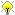[Documentation] [TitleIndex] [WordIndex]

 Note: This tutorial assumes that you have completed the previous tutorials: Controlling turtlesim from Lisp.Please ask about problems and questions regarding this tutorial on answers.ros.org. Don't forget to include in your question the link to this page, the versions of your OS & ROS, and also add appropriate tags.

# Implementing simple plans to move a turtle

Description: In this tutorial you will learn how to implement a simple plan to move a turtle from waypoint to waypoint.

Tutorial Level: INTERMEDIATE

Next Tutorial: Creating Process modules

# Moving the turtle towards a point

To move from one waypoint to the next one, we need to calculate the angular and linear velocity commands. We do that by transforming the goal into the turtle's frame and then calculating the angle towards the goal. Open the file tutorial.lisp of the previous tutorial and add the following code:

```(defun pose-msg->transform (msg)
"returns a transform proxy that allows to transform into the frame given by x, y, and theta of msg."
(with-fields (x y theta) msg
(cl-transforms:make-transform
(cl-transforms:make-3d-vector x y 0)
(cl-transforms:axis-angle->quaternion
(cl-transforms:make-3d-vector 0 0 1)
theta))))

(defun relative-angle-to (goal pose)
"Given a pose as 3d-pose msg and a goal as 3d vector, calculate the angle by which the pose has to be turned to point toward the goal."
(let ((diff-pose (cl-transforms:transform-point
(cl-transforms:transform-inv
(pose-msg->transform pose))
goal)))
(atan
(cl-transforms:y diff-pose)
(cl-transforms:x diff-pose))))

(defun calculate-angular-cmd (goal &optional (ang-vel-factor 4))
"Uses the current turtle pose and calculates the angular velocity
command to turn towards the goal."
(* ang-vel-factor
(relative-angle-to goal (value *turtle-pose*))))```

The function pose-msg->transform returns a transform frame for a given pose message. In relative-angle-to we use it to calculate a diff-pose, which will be the transformed goal pose, and we calculate the relative angle to it from the turtle pose, such that the result may be "20 degrees to the left". (calculate-angular-cmd goal) then just retrieves the current turtle pose from the fluent and multiplies the angle by a factor to make the turtle turn faster.

As an example, if the turtle is at (5, 5) facing the y axis direction ("north"), and the goal is at (1, 2). Then we transform (1, 2) into coordinates relative to the turtles frame, which is 4 "behind" the turtle and 3 to its "left", meaning (-4, 3). The atan function then gives us the angle by which the tutle needs to turn to fact this point. We multiply this by a factor to make the turtle turn faster or slower. Similarly, lin-vel controls if the turtle moves ahead faster or slower.

In order to move the turtle towards a point, we need to continuously recalculate and send the velocity command at a specific rate. The rate must be sufficiently high since the sent command needs to change while the turtle moves. Let's see if our code does what it should do. Enter in the Lisp REPL:

```TUT> (dotimes (i 100)
(send-vel-cmd
1.5 ; linear speed
(calculate-angular-cmd (cl-transforms:make-3d-vector 1 1 0)))
(wait-duration 0.1))```

The code calls send-vel-cmd in a loop 100 times.

The turtle should now move towards the bottom left corner and finally move along a a circle around the goal until the loop finishes.

# Writing a plan to move to a waypoint

Now we need to write a simple plan that recalculates and executes the velocity command until we reach the goal. The easiest way to do that is to construct a fluent over the distance to the goal and use pursue to monitor it in parallel to the control loop. Here the code:

```(def-plan move-to (goal &optional (distance-threshold 0.1))
(let ((reached-fl (< (fl-funcall #'cl-transforms:v-dist
(fl-funcall
#'cl-transforms:translation
(fl-funcall
#'pose-msg->transform
*turtle-pose*))
goal)
distance-threshold)))
(unwind-protect
(pursue
(wait-for reached-fl)
(loop do
(send-vel-cmd
1.5
(calculate-angular-cmd goal))
(wait-duration 0.1)))
(send-vel-cmd 0 0))))```

When we want to use CRAM language features such as pursue, it is good practice to use def-plan instead of defun. The reason is that code in def-plan leads to the creation of a task tree node which makes it transparent for reasoning and for enables online transformation of the code.

The fluent network that we construct looks pretty complicated. We first transform the pose message into a cl-transforms:transform, then we access the translation slot over which we calculate the euclidean distance to the goal.

The pursue block returns as soon as the turtle is within a threshold to the goal. Without an additional message to the controller, the turtle might move on a bit, thus going beyond the goal. Therefore we sent a velocity command afterwards. For robotics it is also important to consider the cases of failures or interrupt of controllers by a developer. To prevent robots from continuing driving into walls. An unwind-protect is therefore generally necessary to stop a robot in all these cases, too, to make a robot fail in a safe state.

The pursue form terminates whenever one of the two body forms terminate. The second form is an endless loop just recalculating and resending the command. The first form terminates as soon as we reached the goal.

To execute CRAM language code we need to either call it from a function that was defined with def-toplevel-plan or we need to wrap it in a top-level form. Let's try it out. Enter the following in the REPL:

```TUT> (top-level
(dolist (goal '((1 1 0) (9 1 0) (9 9 0) (1 9 0) (1 1 0)))
(move-to (apply #'cl-transforms:make-3d-vector goal))))```

The turtle should now move along a rectangle.

2020-02-22 12:38